Processing ......FreeComputerBooks.com Links to Free Computer, Mathematics, Technical Books all over the World

Foundations of Constructive Probability Theory
Top Free JavaScript Books 🌠 - 100% Free or Open Source!
• Title: Foundations of Constructive Probability Theory
• Author(s) Yuen-Kwok Chan
• Publisher: Cambridge University Press; 1st edition (May 27, 2021); eBook (Draft, 2019)
• Hardcover: 550 pages
• eBook: PDF (548 pages)
• Language: English
• ISBN-10/ASIN: 1108835430
• ISBN-13: 978-1108835435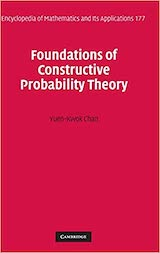Book Description

This book provides a systematic and general theory of probability within the framework of Constructive Mathematics. Using Errett Bishop's work on constructive analysis as a framework, this monograph gives a systematic, detailed and general constructive theory of probability theory and stochastic processes.

It extends the range of constructive mathematics to the arena of probability theory. It can well serve as a parallel introduction into constructive mathematics and rigorous probability theory.

• Yuen-Kwok Chan completed a Ph.D. in Constructive mathematics with Errett Bishop before leaving academia for a career in private industry. He is now an independent researcher in Probability and its applications.
Reviews, Ratings, and Recommendations: Related Book Categories: Read and Download Links:Similar Books:
•Foundations of Infinitesimal Calculus (H. Jerome Keisler)

This monograph is a companion to the Author's textbook. It can be used as a quick introduction to the infinitesimal approach to calculus for mathematicians, as background material for instructors, or as a text for an undergraduate seminar.

•Introduction to Probability, Statistics, and Random Processes

This book introduces students to probability, statistics, and stochastic processes. It provides a clear and intuitive approach to these topics while maintaining mathematical accuracy. The book contains a large number of solved exercises.

•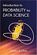Introduction to Probability for Data Science (Stanley Chan)

This book is an introductory textbook in undergraduate probability in the context of data science to emphasize the inseparability between data (computing) and probability (theory) in our time, with examples in both MATLAB and Python.

•Probability Theory: The Logic of Science (E. T. Jaynes)

Going beyond the conventional mathematics of probability theory, this study views the subject in a wider context. It discusses new results, along with applications of probability theory to a variety of problems.

•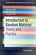Introduction to Random Matrices: Theory and Practice

This is a book for absolute beginners. The aim is to provide a truly accessible introductory account of Random Matrix theory. Most chapters are accompanied by MATLAB codes to guide readers through the numerical check of most analytical results.

•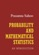Probability and Mathematical Statistics (Prasanna Sahoo)

This book presents an introduction to probability and mathematical statistics for students already having some elementary mathematical background. It blends proven coverage with new innovations to ensure you gain a solid understanding of statistics.

•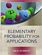Elementary Probability for Applications (Rick Durrett)

This clear and lively introduction to probability theory concentrates on the results that are the most useful for applications, including combinatorial probability and Markov chains. Concise and focused, it is designed for students familiar with basic calculus.

•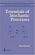Essentials of Stochastic Processes (Rick Durrett)

Stochastic processes have become important for many fields, including mathematical finance and engineering. Written by one of the worlds leading probabilists, this book presents recent results previously available only in specialized monographs.

Book Categories
 :All CategoriesTop Free BooksRecent BooksMiscellaneous BooksComputer EngineeringComputer LanguagesComputer ScienceData Science/DatabasesJava and Java EE (J2EE)Linux and UnixMathematicsMicrosoft and .NETMobile ComputingNetworking and CommunicationsSoftware EngineeringSpecial TopicsWeb Programming
Other Categories